Latest Banking jobs   »

# Quantitative Aptitude Quiz For IRDA AM 2023 -16th May

Q1. Divyam has Rs.40000. He invests some amount in scheme A which offers 30% per annum simple interest and rest of the amount in scheme B which offers 20% compound amount. If after 3 years difference between interest earned from both schemes is Rs.9952, then find amount invested in scheme B.
(a) Rs.24000
(b) Rs.16000
(c) Rs.12000
(d) Rs.18000
(e) Rs.21000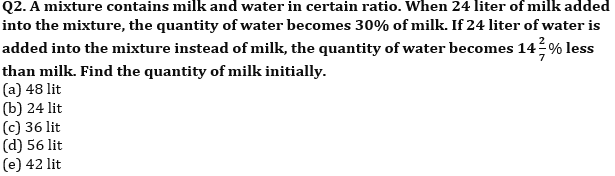Q3. A man purchased two different article A and B at same cost. He sold article A at profit of 20% and article B at 10% loss. If he earned overall profit of Rs.40, then find the cost price of each article.
(a) Rs.300
(b) Rs.500
(c) Rs.400
(d) Rs.800
(e) Rs.600

Q4. In an examination, student A got 65% marks which is 24 more than passing parks while student B got 40% marks and failed by 6 marks. Find the passing percentage marks.
(a) 45%
(b) 40%
(c) 50%
(d) 35%
(e) 30%

Q5. A boat covers a distance D in downstream in 10 hours while in upstream it covers same distance in 40 hours. Speed of boat in still water is 25 kmph, find D.
(a) 300 km
(b) 400 km
(c) 360 km
(d) 240 km
(e) 480 km

Q6. Johny calculates his profit at cost price while Jini at selling price. If cost price is same for all and everyone calculate their profit as 10%. Find ratio of selling price.
(a) 100:111
(b) 10:11
(c) 10:101
(d) 99:100
(e) Cannot be determined

Q7. There are 5 phones cover in a bag which are red & green in color. A cover is drawn at Random. The probability of getting a red cover is 0.6. find green covers.
(a) 1
(b) 2
(c) 3
(d) 4
(e) 5

Q8. In how many ways 5 girls and 3 boys be arranged in a row all facing north such that no boy sit together?
(a) 14400
(b) 1200
(c) 140
(d) 120
(e) 2400

Q9. A man invested Rs.P in three different schemes – A, B and C in the ratio 2 : 1 : 3 respectively. He invested in scheme A at the rate of 10% p.a. at SI for 2 years, in scheme B at the rate of 5% p.a at C.I compounded annually for 2 years and in scheme C at the rate of 6% p.a at CI compounded half yearly for 1 year and received total interest of Rs. 6852. Find the value of P.
(a) Rs.60000
(b) Rs.72000
(c) Rs.48000
(d) Rs.120000
(e) can’t be determined.

Q10. Pipes A, B and C together can fill an empty tank in 10 hours. Pipe A and B together and pipe B and C together can fill the same tank in 12 hours and 20 hours respectively. Find the time taken by pipe B alone to fill the tank?
(a) 25 hours
(b) 20 hours
(c) 15 hours
(d) 30 hours
(e) 40 hours

Solutions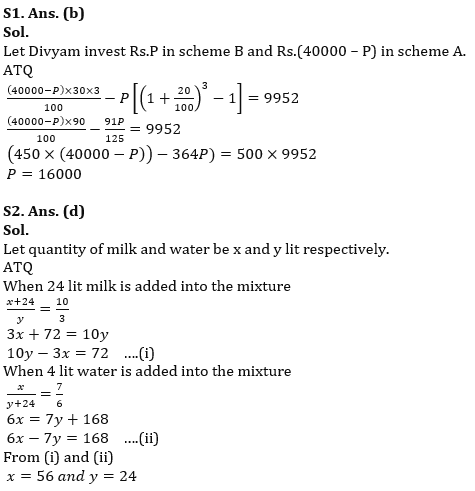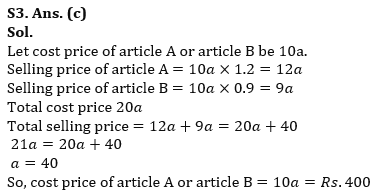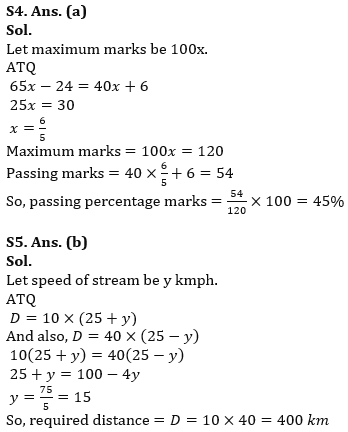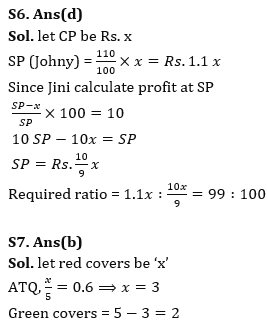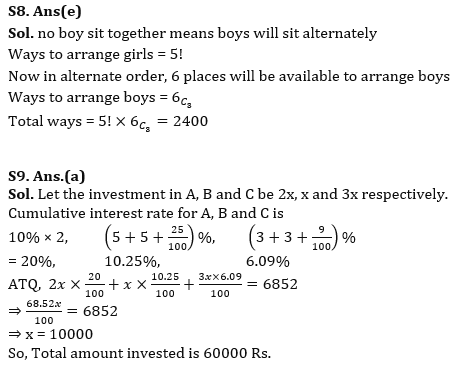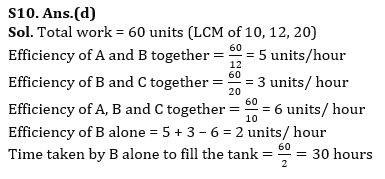## FAQs

### How many sections are there in the IRDAI exam?

There are 4 sections in the IRDAI assistant manager online preliminary exam. They are Reasoning, English Language, General awareness, Quantitative aptitude.

#### Congratulations!Union Budget 2023-24: Free PDF